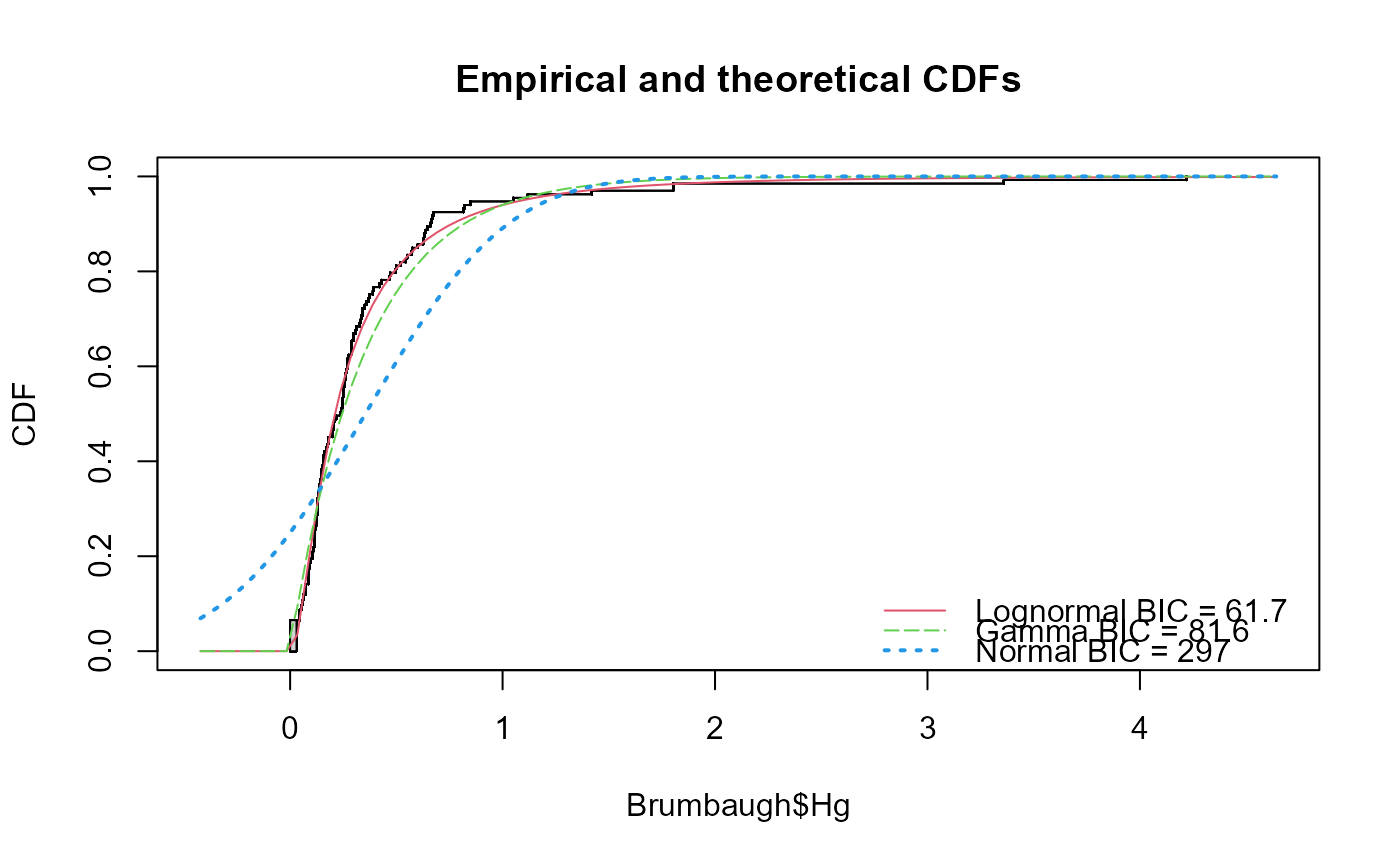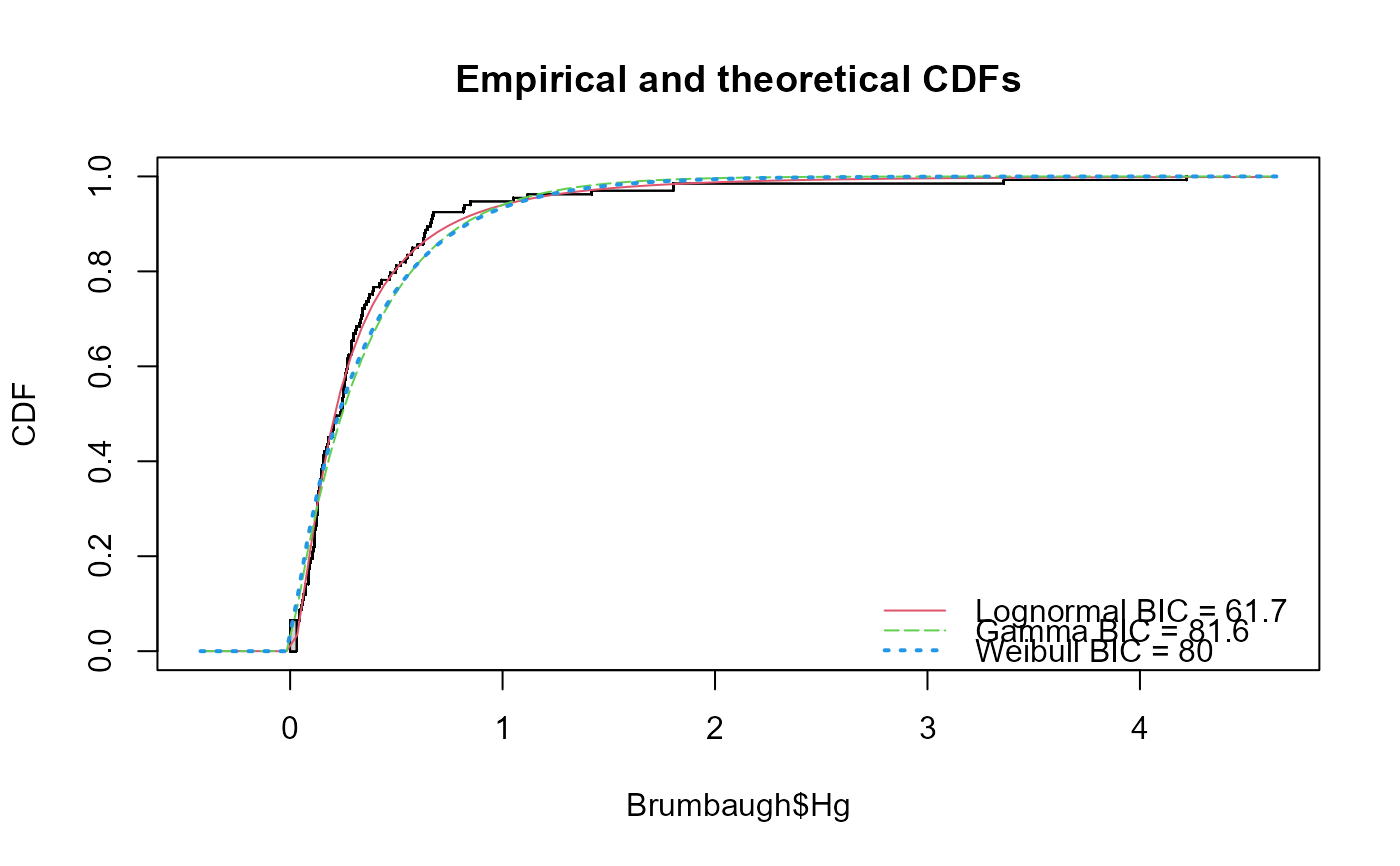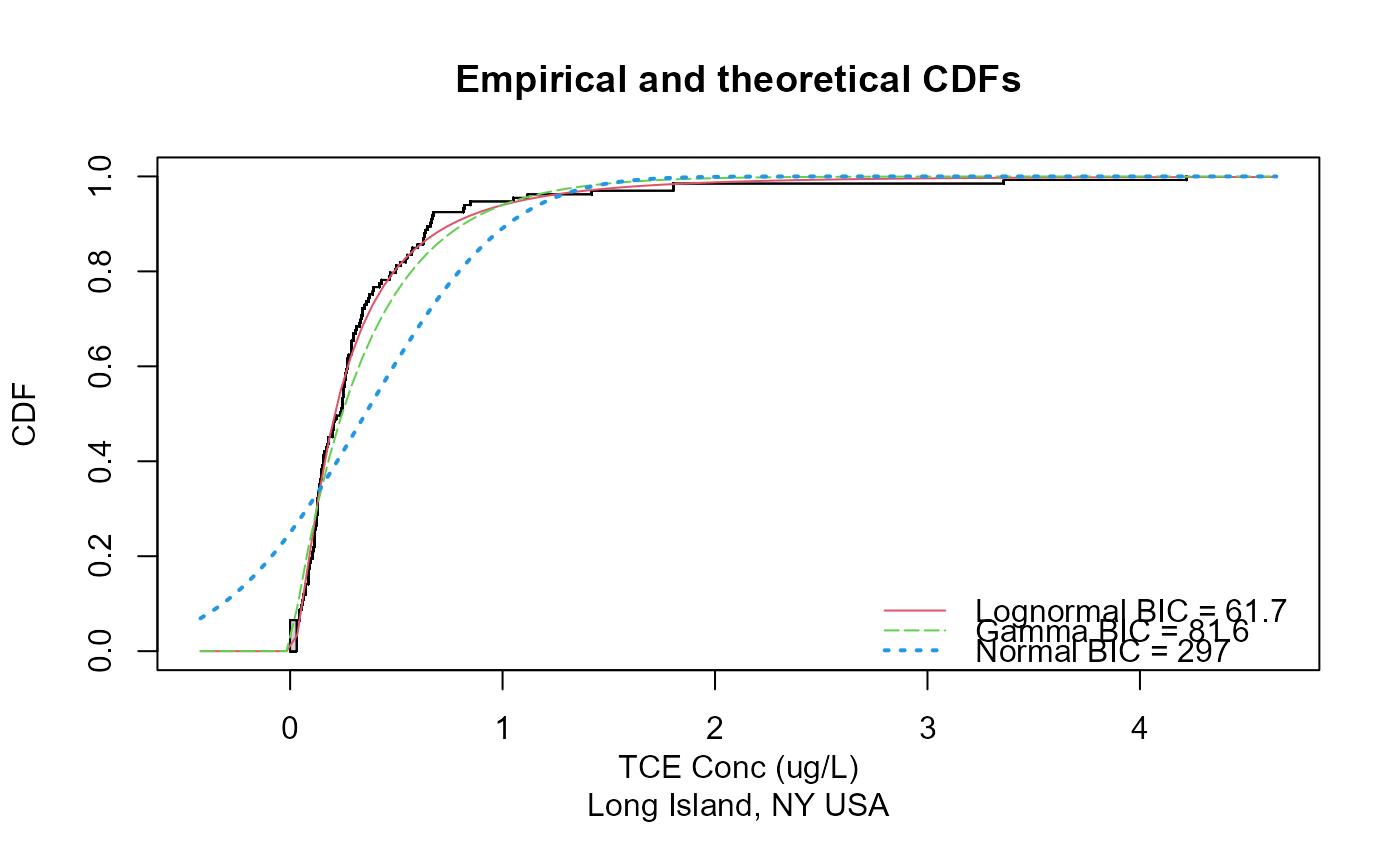Plots the empirical cdf and cdfs of three theoretical distributions, fit by maximum likelihood estimation (MLE).

cenCompareCdfs(x.var, cens.var, dist3 = "norm", Yname = yname)

## Arguments

x.var

The column of y (response variable) values plus detection limits

cens.var

The column of indicators, where 1 (or TRUE) indicates a detection limit in the y.var column, and 0 (or FALSE) indicates a detected value in y.var.

dist3

Name of the third distribution to be plotted, default is norm (normal distribution). Alternate third distribution is weibull(for Weibull distribution). Log-normal and gamma distributions are always used.

Yname

Optional – input text in quotes to be used as the variable name. The default is the name of the y.var input variable.

## Value

prints a plot of the empirical CDFs with BIC value for each distribution.

## Examples


data(Brumbaugh)
cenCompareCdfs(Brumbaugh$Hg,Brumbaugh$HgCen)# With Weibull distribution
cenCompareCdfs(Brumbaugh$Hg,Brumbaugh$HgCen,dist3="weibull")# Using an distribution not supported by this function (yet)
# you will get an error message
if (FALSE) cenCompareCdfs(Brumbaugh$Hg,Brumbaugh$HgCen,dist3="beta")

# With Yname specified
cenCompareCdfs(Brumbaugh$Hg,Brumbaugh$HgCen,Yname="TCE Conc (ug/L)\nLong Island, NY USA")